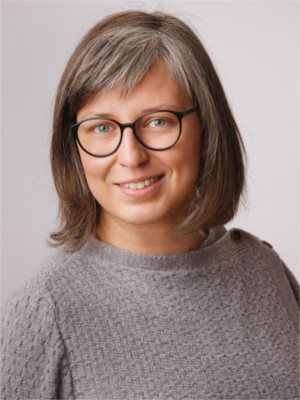## Home

Welcome to my website. I am a mathematician, currently employed as postdoc at the Department of Mathematics at Alpen-Adria-Universität Klagenfurt.

I am tech-savvy and particularly fond of developing and using algorithmic methods in order to formulate and prove mathematical conjectures. My mathematical research interests are

• computer algebra, algebra, number theory, modules and algebras over commutative rings, linear algebra over commutative rings
• integer-valued polynomials, (multivariate) polynomials and polynomials functions over fields as well as possibly non-commutative rings
• theory of non-unique factorizations
• primary decomposition of ideals/modules and associated primes, numbers of associated primes and stabilization index with focus on monomial ideals
• combinatorial commutative algebra, simplicial complices, Stanley-Reisner rings
• integer optimization
• graph theory, centrality notions in graphs
• topological properties of rings and modules
• developing and implementing algorithms for the topics aboveDipl.-Ing.in Dr.in techn. Roswitha Rissner

: +43 463 2700 3149

Department of Mathematics (Room N.2.25)